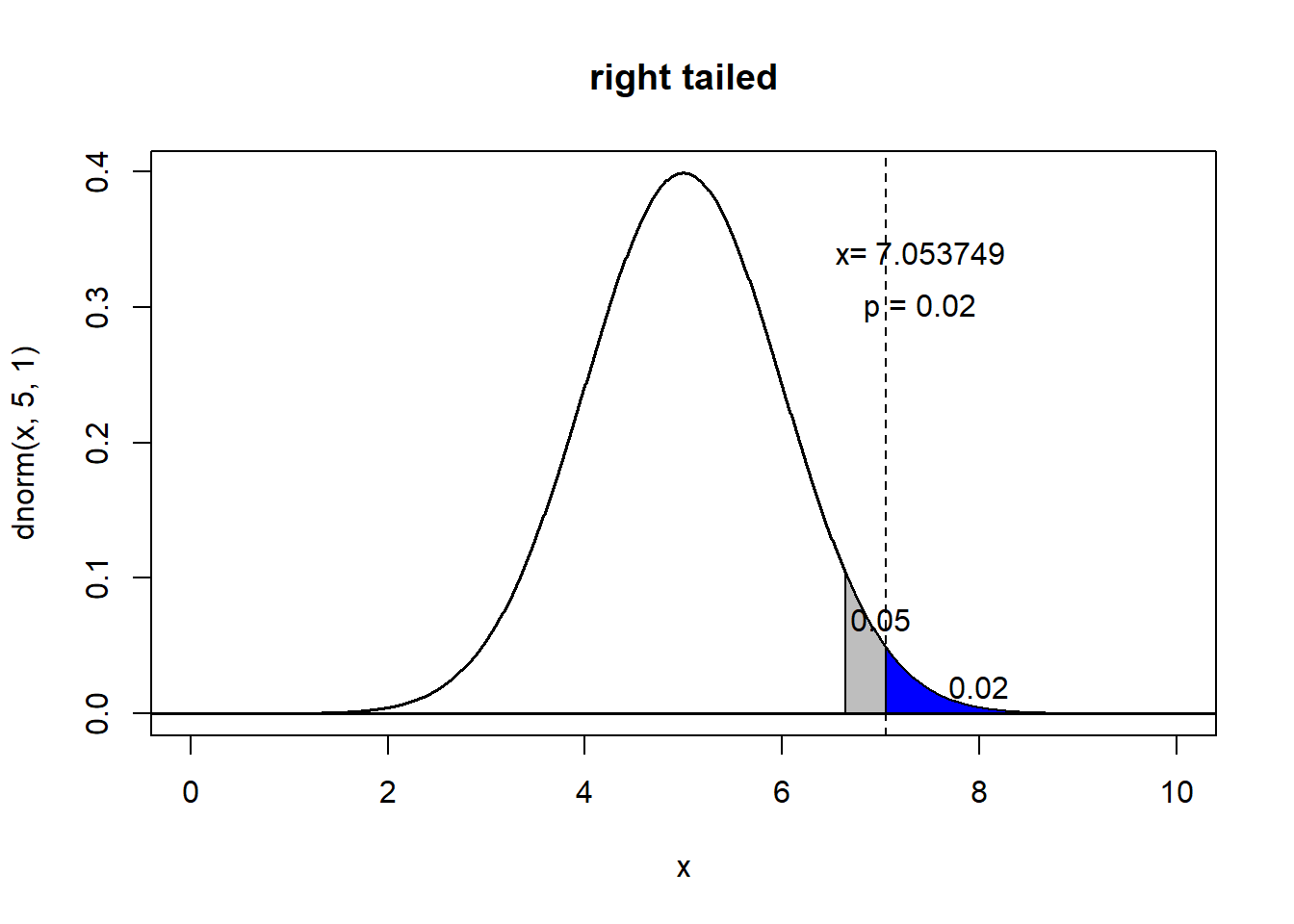# 2. 统计假设检验

## 2.1 统计假设(statistical hypothesis)

• 学校所有学生年龄的平均数是23.4岁
• 学校学生中女生的比例是76%
• 学校中学生的身高H，近似为正态分布

## 2.2 统计假设检验

• 零假设使用$$H_0$$表示
• 备选假设使用$$H_1$$表示

• 学校所有学生年龄的平均数不是23.4岁
• 学校所有学生年龄的平均数大于23.4岁
• 学校所有学生年龄的平均数是27.4岁

## 2.4 统计假设检验的几个例子

2. 检测是否男性比女性更容易受到噩梦的干扰
3. 验证月圆对行为的影响
4. 确定蝙蝠可以通过回声定位昆虫的距离
5. 研究医院的毛毯会不会导致更多的感染
6. 选择阻止吸烟最好的办法

# 3. 两类错误

• 第一类错误(Type I error): 在零假设正确的时候, 错误地拒绝了零假设.
• 第二类错误(Type II error): 在零假设错误的时候, 没能拒绝零假设.

• Pr[Type I error] = $$\alpha$$
• Pr[Type II error] = $$\beta$$

## 3.1 第一类错误的概率

$$\alpha$$值指的是犯第一类错误的概率, 这个值也被称为是显著性水平(significant level). 这个值是在样本收集前指定的, 根据不同的问题可以指定不同的$$\alpha$$值。 最常用的$$\alpha=0.05$$. 因为$$\alpha$$表示在零假设为真的情况下拒绝零假设的概率, 而当零假设为真的时候, 只有样本值显著与零假设有差异, 才会拒绝零假设, 也就是说, $$\alpha$$表示了在概率分布中最靠外的地方. 因此就有以下三种情况:## 3.2 第二类错误的概率

$$\beta$$表示第二类错误发生的概率, 因此$$1-\beta$$就表示不犯第二类错误的概率, 因为一般来讲, 避免第二类错误更加重要一些, 所以1-$$\beta$$的值就受到更多关注, 把它称为检验的势(Power). 即一个检验的势, 指的是在零假设为假并且判断正确的概率. 检验的势越高越好, 强势检验也被称为高效率检验.

# 4. p-value

p值可以这样给出:

• $$Pr(X \geq x|H)$$ for right tail event
• $$Pr(X \leq x|H)$$ for left tail event
• 2 $$min\{Pr(X \geq x|H), \; Pr(X \leq x|H)\}$$ for double tail event

p值越小, 出现在零假设外极端值的概率也就越小。因此在测试中, 这样的样本本应该极不可能出现的, 却被观察到了, 也就说明零假设是可以拒绝的。即p值越小，越能拒绝零假设

$$Pr(p\leq \alpha | H)$$时, 拒绝零假设. 所以有: $Pr(Reject \; H) = Pr(p\leq \alpha | H) = \alpha$x = c(172,180,179,160,175)
t.test(x, m=165, alternative = "greater")
##
##  One Sample t-test
##
## data:  x
## t = 2.2795, df = 4, p-value = 0.04241
## alternative hypothesis: true mean is greater than 165
## 95 percent confidence interval:
##  165.5313      Inf
## sample estimates:
## mean of x
##     173.2

1. $$t = \frac{\bar{x}-\mu}{\frac{s}{\sqrt{n}}}$$ 所以代入，求得$$t = ^{(mean(x) - 165) \times \sqrt{5} }/ sd(x)= 2.2795$$
2. 按照n-1建立t分布，即t(4)
3. 用t值去对应t分布的概率1-pt(t, 4)=0.04241492, 结果与直接使用t.test结果一致。

# 5. 统计假设检验的一般流程

1. 对于未知的事实，提出一个最初的研究假设。
2. 建立零假设备选假设，这一步非常关键，错误的零假设会导致结果完全错误。正确指定零假设的关键在于零假设总是会指定一个参数 等于 某个值
3. 考虑样本的统计学假设，比如抽样的独立性，比如样本的分布等。样本的数据同样很关键，否则可能得出完全相反的结论。比如错误地拒绝零假设等。
4. 考虑选择检验统计量(test statistic) T, 比如T统计量。不同的检验统计量对应了不同的检验，应用不同的比较上。例如单样本z检验，检验统计量就是z值; 单样本t检验，检验统计量就是t值。
5. 推导零假设下检验统计量的分布，在标准情况下一般是t分布或者正态分布。如上一个例子中，第一步做了t值的计算，第二步就说明样本分布遵循t分布(因为原概率分布为正态分布，而样本大小比较小并且对原分布的标准差未知)。
6. 选择显著性水平(significance level, $$\alpha$$)，一般都是5%或者1%
7. 按照检验统计量(test statistics)的分布来查看对应的检验统计量的值$$t_{obs}$$，是否落入拒绝域，拒绝零假设